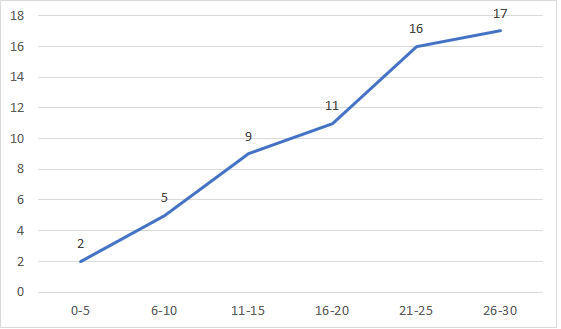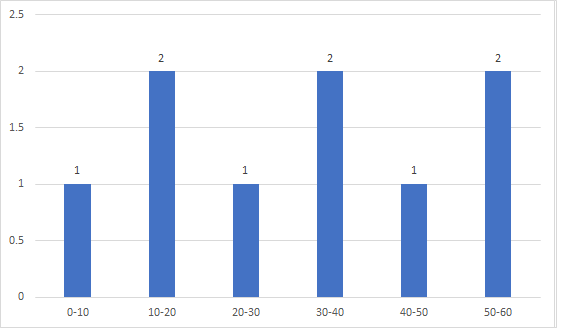# Definition of an Ogive Graph in Statistics

Instructions:

question 1 of 3

### In this ogive plot, how many observations (y-axis) do we expect for all participants between 0 and 20 years of age (x-axis)?Create Your Account To Take This Quiz

As a member, you'll also get unlimited access to over 79,000 lessons in math, English, science, history, and more. Plus, get practice tests, quizzes, and personalized coaching to help you succeed.

Try it risk-free for 30 days. Cancel anytime

### 1. Which of the following graphs is the ogive graph for the following set of observations?### 2. Ogive graphs are often also referred to as _____ frequency plots.

Create your account to access this entire worksheet
Quizzes, practice exams & worksheets
Certificate of Completion
Create an account to get started

Answer these questions to see what you know about ogive charts. You will need to explain how to use an ogive plot and how observations are measured.

## Quiz & Worksheet Goals

In this set of questions, you'll measure your understanding of:

• Using a ogive graph to find the number of observations between two points
• Other names for ogive graphs
• Where different limits are presented on an ogive plot

## Skills Practiced

• Reading comprehension - ensure that you draw the most important information from the material, such as another name for an ogive graph
• Problem solving - use acquired knowledge to solve ogive graph and plot practice problems
• Information recall - access the knowledge you've gained regarding how observations are measured on an ogive graph

• The uses of an ogive chart
• Frequency plots
• Examples of ogive plots
Final ExamMath for Kids
Status: Not Started
Chapter ExamWorking with Data for Elementary School
Status: Not Started

Support